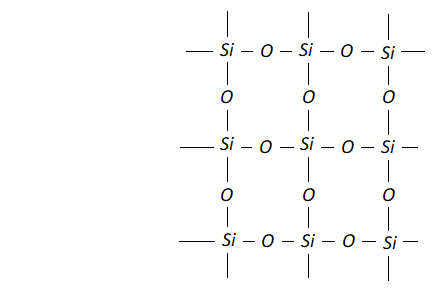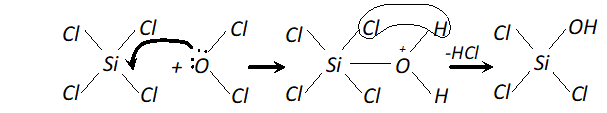Download the eSaral app and start learning from Kota's top IITians and doctors.

# Carbon Family | Question Bank for Class 11 Chemistry

Q. Is carbon dioxide poisonous or not ?
Ans. Carbon dioxide is not poisonous.
Q. What is dry ice ? Why is it so called ?
Ans. Solid carbon dioxide gas is called dry ice, solid carbon dioxide can sublime to the gaseous state without passing through the liquid state and therefore, it is called dry ice.
Q. What is state of hybridisation of carbon in (i) $\mathrm{CO}_{3}^{2-}$ (ii) diamond (iii) graphite? [NCERT]
Ans. The hybridisation of carbon in (i) $\mathrm{CO}_{3}^{2-}$ is $s p^{2}$ (ii) in diamond is $s p^{3}$ and in (iii) graphite is $\mathrm{sp}^{2}$
Q. Name the isotopes of carbon used for radio carbon dating and for international standard for atomic mass.
Ans. Isotope of carbon used for radio carbon dating is 14 C. Isotope of carbon used for international standard for atomic mass is $12 \mathrm{C}$.
Q. What is allotropy ?
Ans. Allotropy. "The existence of an element in two or more different forms which have different physical properties but same chemical properties, is called allotropy and the forms are called allotropes." The allotropic forms of carbon are: (i) Crystallined forms - Diamond, graphite. (ii) Non-Crystallined forms - Charcoal, lamp-black.
Q. Explain the differences in the properties of diamond and graphite on the basis of their structures. [NCERT]
Ans. Diamond is very hard crystalline solid because each carbon is linked to four other carbon atoms tetrahedrally by using $s p^{3}$ hybrid orbitals giving rise to a rigid three dimensional network of carbon atoms. It does not conduct electricity because it does not have free electrons. Graphite has structure in which each carbon is bonded to three other carbon atoms to form a hexagonal sheet. Each 'CI atoms forms three sigma bonds by means of $s p^{2}$ hybrid orbitals. The unhybridised $p$ -orbitals of each carbon atoms having electrons overlaps with $p$ -orbitals of other carbon atoms to form $\pi$ -bonds. The hexagonal layers are held together by weak Vander Wall's force of attraction, that is why it is soft and slippery and used as lubricant. It is conductor of electricity because -electrons within layer are free to move and this accounts for electrical conductivity.
Q. What is the oxidation state of carbon in each of the followingcompounds? ( i ) $\mathrm{CO}$ (ii) $\mathrm{HCN}$ (iii) $\mathrm{H}_{2} \mathrm{CO}_{3}$ (iv) $\mathrm{CaC}_{2}$
Ans. (i) 2 (ii) 2 (iii) 4 (iv) –1
Q. Why carbon forms covalent compounds whereas lead forms ionic compounds ?
Ans. - Carbon cannot lose electrons to form $C^{4+}$ because the ionization energy required is very high. It cannot gain electrons to form $C^{4-}$ because it is energetically not favourable. Hence $C$ forms covalent compounds. Down the group, the $I . E .$ decreases. $P b$ being the last element has so low, I.E. that it can lose electrons to form ionic compounds.
Q. What happens when : (i) Quick lime is heated with coke? (ii) Carbon monoxide reacts with $C l_{2} ?$ (iii) Plants absorb $\mathrm{CO}_{2} ?$
Ans. (i) Calcium carbide and Carbon monoxide are formed. $\mathrm{CaO}+3 \mathrm{C} \stackrel{\text { heat }}{\longrightarrow} \mathrm{CaC}_{2}+\mathrm{CO}$ (ii) Phosgene gas is formed. $\mathrm{CO}+\mathrm{Cl}_{2} \longrightarrow \mathrm{COCl}_{2}$ [Phosgene gas (Carbonyl Chloride)] (iii) They form glucose and starch.
Q. What are carbides? How are they classified? Give one example of each type of carbides.
Ans. Compounds of electropositive elements with carbon are called carbides. There are three types of Carbides: (i) Ionic Carbide : Most electropositive metals form ionic carbide, e.g., calcium carbide. (ii) Covalent Carbide : Less electropositive metals and nonmetals form covalent carbides, e.g., silicon carbide, boron carbides. (iii) Interstitial Carbides : Those carbides which are formed by transition metals in which carbon atoms occupy interstitial voids, $e . g ., W C(\text { Tungsten Carbide })$
Q. Explain the following: (i) Silanes are few in number while alkanes are large in number. (ii) Conc. $H N O_{3}$ can be stored in aluminium container but it cannot be stored in zinc container. (iii) Burning magnesium continues to burn while burning sulphur gets extinguished when dropped in gas containing $\mathrm{NO}$ (iv) Aqueous solution of carbonates and bicarbonates of alkali metals is alkaline.
Ans. (i) Carbon has the maximum tendency for catenation due to stronger $C-C \quad\left(353.5 \mathrm{kJ} \mathrm{mol}^{-1}\right)$ and hence it forms large number of alkanes. silicon, on the other hand, bonds has much lesser tendency for catenation due to weak $S i-S i \quad\left(200 k J \mathrm{mol}^{-1}\right)$ and hence it forms only few silanes (ii) In presence of conc. $H N O_{3}, A l$ becomes passive, due to a thin protective layer of its oxide $\left(A l_{2} O_{3}\right)$ which is formed on its surface which prevents the further action between the metal and the acid. Therefore $A l$ containers can be used for storing conc. However, it cannot be stored in zinc vessels because zinc reacts with $\mathrm{HNO}_{3}$. $\mathrm{Zn}+4 \mathrm{HNO}_{3} \longrightarrow \mathrm{Zn}\left(\mathrm{NO}_{3}\right)_{2}+2 \mathrm{NO}_{2}+2 \mathrm{H}_{2} \mathrm{O}$ (iii) Heat evolved during burning of $M g$ is enough to decompose $N O$ to $\mathrm{N}_{2}$ and $\mathrm{O}_{2} .$ The $\mathrm{O}_{2}$ thus, produced support combustion of $M g .$ In contrast, the heat produced during burning of $S$ is not quite sufficient to decompose $N O .$ As a result, sulphur stops burning. (iv) The alkaline nature of aqueous solution is due to the hydrolysis of these salts. $\underset{\text { Bicarbonates }}{H C O_{3}^{-}}+H_{2} O \rightleftharpoons H_{2} C O_{3}+\overline{O H}$ $\underset{\text { Cattonates }}{C O_{3}^{2-}}+H_{2} O \rightleftharpoons H C O_{3}^{-}+\overline{O H}$
Q. Explain the following: (i) $\quad \mathrm{SiO}_{2}$ is solid but $\mathrm{CO}_{2}$ is a gas at room temperature. (ii) $\quad \mathrm{SiCl}_{4}$ can be easily hydrolysed but $\mathrm{CCl}_{4}$ does not hydrolyse.
Ans. (i) Carbon is able to form $p \pi-p \pi$ bond with $O$ atom and constitute a stable non-polar molecule $O=C=O .$ Due to weak interparticle forces its boiling point is low and it is a gas at room temperature. Si$,$ on the other hand is not able to form bond with $O$ atoms because of its relatively large size. In order to complete it octet sillicon is linked to four $O$ atoms around it by sigma bonds and thus it constitutes network structure which is responsible for its solid state.(ii) In $\mathrm{SiCl}_{4},$ the silicon atom has empty 3 dorbitals in its valence shell which can accept an electron pair from $H_{2} O$ molecule resulting the co-ordinate bond. The co-ordinated molecule loses a molecule of $H C l$ and as a result, a chlorine atom gets replaced by an $O H$ group as shown.In the same way, all the four $C l$ atoms are replaced by $O H$ group and the molecule gets completely hydrolyse. Carbon atom, on the other hand does not have vacant $d$ -orbitals in 2nd level. Hence, it cannot accept electron pair form $H_{2} O$ molecule and therefore, $\mathrm{CCl}_{4}$ is not hydrolysed.